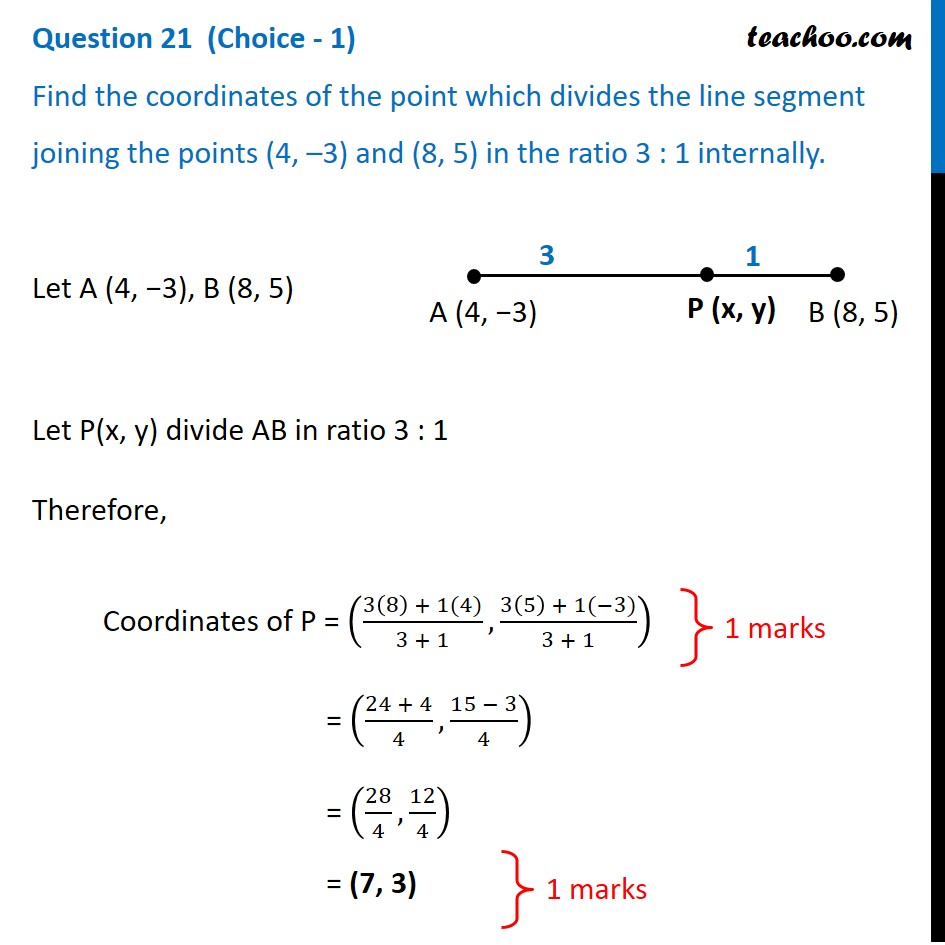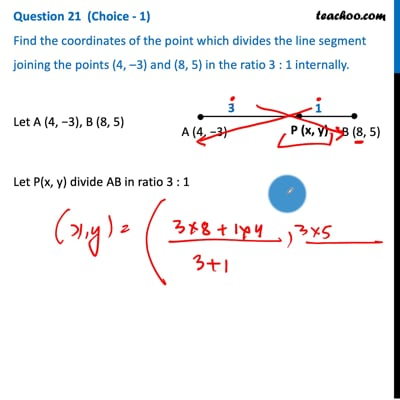CBSE Class 10 Sample Paper for 2021 Boards - Maths Basic

Class 10
Solutions of Sample Papers for Class 10 Boards

## Find the coordinates of the point which divides the line segment joining the points (4, –3) and (8, 5) in the ratio 3 : 1 internally.This video is only available for Teachoo black users

Introducing your new favourite teacher - Teachoo Black, at only ₹83 per month

### Transcript

Question 21 (Choice - 1) Find the coordinates of the point which divides the line segment joining the points (4, –3) and (8, 5) in the ratio 3 : 1 internally.Let A (4, −3), B (8, 5) Let P(x, y) divide AB in ratio 3 : 1 Therefore, Coordinates of P = ((3(8) + 1(4))/(3 + 1),(3(5) + 1(−3))/(3 + 1)) = ((24 + 4)/4,(15 − 3)/4) = (28/4,12/4) = (7, 3) 1 marks 1 marks